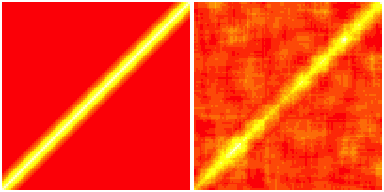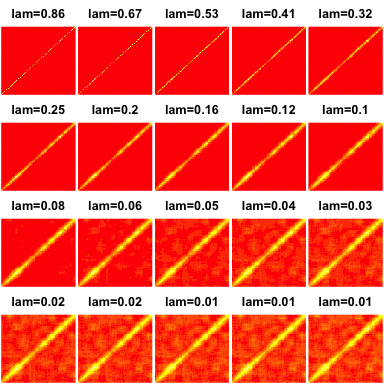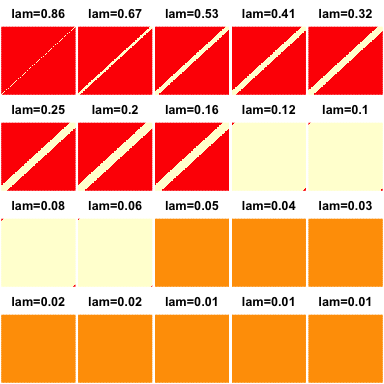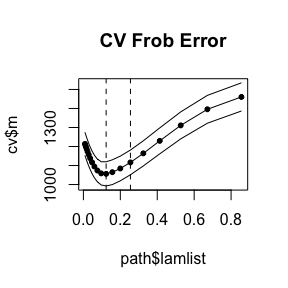The hierband package implements the convex banding procedure for covariance estimation that is introduced in Bien, Bunea, Xiao (2015) Convex Banding of the Covariance Matrix.’’ To appear in JASA. This document shows how the package can be used.

## Generating some data

We start by generating a $$n \times p$$ data matrix, whose rows are independent draws from a multivariate normal distribution with covariance matrix $$\Sigma$$. We take $$\Sigma$$ to be a $$K$$-banded matrix.

library(hierband)
set.seed(123)
p <- 100
n <- 50
K <- 10
true <- ma(p, K)
x <- matrix(rnorm(n*p), n, p) %*% true$A S <- cov(x) Let’s look at the true covariance matrix, $$\Sigma$$, and the sample covariance matrix, $$S$$: par(mfrow=c(1,2),mar= rep(0.1, 4)) image(true$Sig,axes=F)
image(S, axes=F)## Generating solutions along the convex banding solution path

The functiont hierband takes the sample covariance matrix and returns a banded matrix. It depends on a tuning parameter $$\lambda$$ that controls the bandwidth of the estimated matrix (which we call $$\hat P_\lambda$$). The function hierband.path gets $$\hat P_\lambda$$ along a (log-linear) grid of $$\lambda$$ values. While a user may supply this grid of $$\lambda$$ values (using the argument lamlist), a default grid is used that starts at a value of $$\lambda$$ for which $$\hat\Sigma_\lambda$$ is a diagonal matrix.

library(hierband)
path <- hierband.path(S)
## 1234567891011121314151617181920

Let’s look at the $$\hat P_\lambda$$’s generated:

par(mfrow = c(4, 5), mar = 0.1 + c(0, 0, 2, 0))
for (i in seq_along(path$lamlist)) image(path$P[, , i], axes = F,
main = sprintf("lam=%s", round(path$lamlist[i], 2)))Sometimes one finds that all solutions are sparse, meaning that the default grid is not wide enough, i.e., we should include smaller values of $$\lambda$$. This can be adjusted with the parameter flmin (which is the ratio between the largest and smallest $$\lambda$$ values in the grid); also, nlam can be used to control the number of grid points used (the default is 20). Let’s check whether we are getting the full range of sparsity levels: par(mfrow = c(4, 5), mar = 0.1 + c(0, 0, 2, 0)) for (i in seq_along(path$lamlist))
image(path$P[,,i] != 0, axes = F, main = sprintf("lam=%s", round(path$lamlist[i], 2)))In this case, we see that we are getting a full spectrum of bandwidths (the last few images show that $$\hat P_\lambda$$ is completely dense).

## Selecting the tuning parameter

To select a value for $$\lambda$$, the hierband package provides a cross validation function. The default loss function used is squared Frobenius norm, $$\|\hat P_\lambda-\Sigma\|_F^2$$; however, other loss functions can be passed through the errfun argument.

cv <- hierband.cv(path, x)
## 1234567891011121314151617181920
## 1234567891011121314151617181920
## 1234567891011121314151617181920
## 1234567891011121314151617181920
## 1234567891011121314151617181920
fit <- hierband(S, lam = cv$lam.best) plot(path$lamlist, cv$m, main = "CV Frob Error", type="o", ylim = range(cv$m - cv$se, cv$m + cv$se), pch = 20) lines(path$lamlist, cv$m + cv$se)
lines(path$lamlist, cv$m - cv$se) abline(v = path$lamlist[c(cv$ibest, cv$i.1se.rule)], lty = 2)The two dotted lines correspond to the selected value of $$\lambda$$ using either the one-standard error rule ($$\lambda=0.2547905$$) or the minimizer of the curve ($$\lambda=0.1231385$$).

## How well did we do?

Since the data was simulated, we know the true covariance matrix $$\Sigma$$ and can check how close our estimate is to the truth and how this compares to the sample covariance matrix.

sqrt(mean((fit - true$Sig)^2)) ##  0.08560086 sqrt(mean((S - true$Sig)^2))
##  0.1372773

We find that in this example we get 0.62 closer than the sample covariance matrix. Of course, this is on the basis of a single iteration. In the paper, we averaged over many iterations to get mean squared errors.

## Acknowledgment

The development of this vignette (and R package) was supported by National Science Foundation grant DMS-1405746.